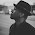Tuesday, December 30, 2014

Why does an electronic load draw a pulse of current when a voltage is initially applied?

We recently had a customer contact us about one of our electronic loads. He had a solid state switch in series with a fixed output voltage source (for example, 50 V) and set the load to a fixed current (for example, 1 A). He used a current probe and a scope to observe the current flowing into the electronic load. When the switch changed from open to closed he saw a pulse of current flowing into the load that was significantly higher than the load set value before the load settled to the set value of 1 A. He was wondering if this was normal. It is normal. Here’s why:

The electronic loads have a snubber network across their input terminals. The snubber typically consists of a resistor in series with a capacitor. For example, the Keysight N3304A electronic load has 2.2 uF in series with about 2 ohms. The snubber network is there to maintain stability on the load input for all settings and operating modes. When the customer’s switch was closed, the initially discharged capacitor in the snubber pulled a pulse of current to begin charging. If the dV/dt of the load input voltage waveform was infinitely fast, the cap would initially look like a short and the initial current pulse would be limited by the resistor as I = V/R. In this example, the current pulse would have been 50 V / 2 ohms = 25 A. But he was seeing a much smaller current pulse: around 2.4 A instead of 25 A, but still higher than the expected set value of 1 A. This means the dV/dt was not infinite (the solid state switch had a finite risetime). In this case, the current pulse would be limited by the dV/dt of the input voltage waveform.

As an example, see Figure 1 below showing the input voltage and input current for an N3304A load. The voltage rises from 0 V to 50 V over about 75 us and the fastest part of the risetime is about 1V/us. Since I = C dV/dt, and for this electronic load, C = 2.2 uF, the peak of the current is calculated to be 2.2 uF x 1V/us = 2.2 A. The plot shows it to be about 2.4 A, so this is close to the expected peak value. As the dV/dt of the input voltage slows down, the current drops from its peak and approaches zero amps as the dV/dt slows to zero (horizontal). (Note that in the plot below, the load was set for zero current.)

So you can see that the current flowing into the input of an electronic load may not be simply the DC setting you expect. If you apply a dynamic voltage waveform to the input, the RC snubber network will also draw some current for a short time until the voltage applied to the load input stabilizes. There is another factor involved here that is worth mentioning but I will not cover in detail in this post since it is a secondary effect in this case. In this customer’s situation, the load was set for 1 A and initially had no voltage on it (his solid state switch was open). The load was trying to draw current by turning on its input FETs, but there was no voltage applied, so the load went to an unregulated state. When the voltage finally appeared (the solid state switch was closed), the FETs that were turned on hard had to recover and take a finite amount of time to begin regulating the set current. This effect can also contribute to brief, temporary unexpected current draw by the load when a voltage is suddenly applied to the input.

1 comment:

1.If you need help to write a research essay for college, these tips will make it easier for you to write it.